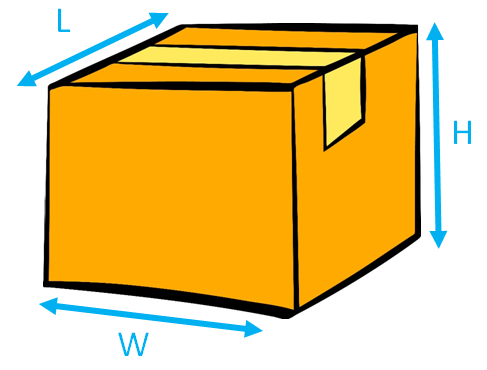We Deliver Happiness

# Explaining shipment weight

Simply, courier companies charge shipment costs on actual weight or volumetric weight (whichever is higher). Actual weight is the weight of the parcel.

It is important to understand as the shipments cost do get affected by volume of the parcel if the volume weight is more than the actual weight of the parcel. To be sure, please see below the explanation of how the volumetric weight is calculated by the courier companies.

Volumetric or dimensional weight -

Volumetric weight is calculated on parcel size rather than the actual weight of the item. This is done by the courier companies to ensure they charge accurately and for the space parcel will take on the aircraft. This is a common practice around the world with courier companies.

Formula to calculate the volumetric weight in KG

Length (cm) x Height (cm) x Width (cm)   =   Volumetric Weight
5000Example:

Parcel 1:
(60cm x 20cm x 20cm) / 5000
Volumetric Weight = 4.8kg
Actual Weight = 7.83kg

Actual weight will be charged -

Parcel 2:
(90cm x 60cm x 30cm) / 5000
Volumetric Weight = 32.4kg
Actual Weight = 21.90kg

Volumetric weight will be charged -